﻿ 太空电梯力学特性分析 Study on Mechanical Characteristics of Space Elevator

Journal of Aerospace Science and Technology
Vol. 06  No. 03 ( 2018 ), Article ID: 27049 , 8 pages
10.12677/JAST.2018.63005

Study on Mechanical Characteristics of Space Elevator

Man Ru1, Mingwei Yin2

1The High School Affiliated to Renmin University of China, Beijing

2Department of Aerospace Engineering, Tsinghua University, Beijing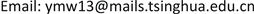Received: Sep. 8th, 2018; accepted: Sep. 23rd, 2018; published: Sep. 30th, 2018ABSTRACT

Space elevator is a new way to transporting loads to space at a low cost, which is one of the most important development trends. Based on the method of theoretical mechanics, this paper gives a discussion of the relationship between the location of the space station and the length of the elevator. For some designs of the space elevator, this paper discusses the distribution of stress and points at the position and value of the largest stress. Finally, the lowest energy and the lateral controlling impulse are calculated when a unit load is transported to space.

Keywords:Space Elevator, Mechanical Characteristics, Stress Distribution, Controlling Impulse

1中国人民大学附属中学，北京

2清华大学航天航空学院，北京Copyright © 2018 by authors and Hans Publishers Inc.1. 引言

2. 太空站位置与电梯长度的分析

2.1. 太空站在地球同步轨道上

${F}_{1}=\underset{{R}_{e}}{\overset{{R}_{0}+L}{\int }}\frac{GM\lambda \text{d}r}{{r}^{2}}$ (1)

${F}_{2}=\underset{{R}_{e}}{\overset{{R}_{0}+L}{\int }}{\omega }^{2}r\lambda \text{d}r$ (2)

${\omega }^{2}{R}_{0}=\frac{GM}{{R}_{0}^{2}}$ (3)

$L=\sqrt{\frac{2{R}_{0}^{3}}{{R}_{e}}+\frac{1}{4}{R}_{e}^{2}}-\frac{1}{2}{R}_{e}-{R}_{0}$ (4)

${\lambda }_{1}$ 为太空电梯从地面到太空站区间的线密度， ${\lambda }_{2}$ 为太空电梯配重段的线密度，令 $k={\lambda }_{2}/{\lambda }_{1}$ 。地球对太空电梯的引力F1为：

${F}_{1}=\underset{{R}_{e}}{\overset{{R}_{0}}{\int }}\frac{GM{\lambda }_{1}\text{d}r}{{r}^{2}}+\underset{{R}_{0}}{\overset{{R}_{0}+L}{\int }}\frac{GM{\lambda }_{2}\text{d}r}{{r}^{2}}$ (5)

${F}_{2}=\underset{{R}_{e}}{\overset{{R}_{0}}{\int }}{\omega }^{2}r{\lambda }_{1}\text{d}r+\underset{{R}_{0}}{\overset{{R}_{0}+L}{\int }}{\omega }^{2}r{\lambda }_{2}\text{d}r$ (6)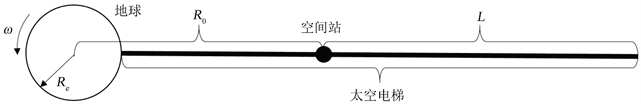Figure 1. The diagram of space elevator

 (7)

2.2. 太空站不在地球同步轨道上

${F}_{3}=m\left({\omega }^{2}{R}_{0}-\frac{GM}{{R}_{0}^{2}}\right)$ (8)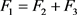可以得到一下关于电梯配重段长度L的约束：

$\begin{array}{l}\frac{1}{2}k{L}^{3}+\frac{3}{2}k{R}_{0}{L}^{2}+\left[k{R}_{0}^{2}+\frac{1}{2}\left({R}_{0}^{2}-{R}_{e}^{2}\right)+{R}_{s}\left({R}_{0}-\frac{{R}_{G}^{3}}{{R}_{0}^{2}}\right)-\frac{{R}_{G}^{3}}{{R}_{e}}-\frac{k{R}_{G}}{{R}_{0}}+\frac{{R}_{G}^{3}}{{R}_{0}}\right]L\\ +\frac{1}{2}\left({R}_{0}^{2}-{R}_{e}^{2}\right){R}_{0}+{R}_{s}\left({R}_{0}-\frac{{R}_{G}^{3}}{{R}_{0}^{2}}\right){R}_{0}+{R}_{G}^{3}-\frac{{R}_{G}^{3}{R}_{0}}{{R}_{e}}=0\end{array}$ (9)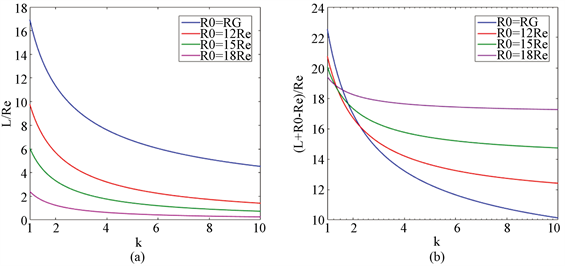Figure 2. The relationship between the length of the whole elevator, the length of the counterweight elevator and the location of space station, the ratio of different materials’ density

3. 太空电梯径向方向应力分布

$F\left(R\right)=\left\{\begin{array}{l}\underset{{R}_{e}}{\overset{R}{\int }}\left(\frac{GM}{{r}^{2}}-{\omega }^{2}r\right){\lambda }_{1}\text{d}r,\text{\hspace{0.17em}}\text{\hspace{0.17em}}\text{\hspace{0.17em}}\text{\hspace{0.17em}}\text{\hspace{0.17em}}\text{\hspace{0.17em}}\text{\hspace{0.17em}}\text{\hspace{0.17em}}\text{\hspace{0.17em}}\text{\hspace{0.17em}}\text{\hspace{0.17em}}\text{\hspace{0.17em}}\text{\hspace{0.17em}}\text{\hspace{0.17em}}\text{\hspace{0.17em}}\text{\hspace{0.17em}}\text{\hspace{0.17em}}\text{\hspace{0.17em}}\text{\hspace{0.17em}}\text{\hspace{0.17em}}\text{\hspace{0.17em}}\text{\hspace{0.17em}}\text{\hspace{0.17em}}\text{\hspace{0.17em}}\text{\hspace{0.17em}}\text{\hspace{0.17em}}\text{\hspace{0.17em}}\text{\hspace{0.17em}}\text{\hspace{0.17em}}\text{\hspace{0.17em}}\text{\hspace{0.17em}}\text{ }\text{ }\text{\hspace{0.17em}}\text{\hspace{0.17em}}\text{\hspace{0.17em}}\text{\hspace{0.17em}}\text{\hspace{0.17em}}\text{\hspace{0.17em}}\text{\hspace{0.17em}}\text{\hspace{0.17em}}\text{\hspace{0.17em}}\text{\hspace{0.17em}}\text{\hspace{0.17em}}\text{\hspace{0.17em}}\text{\hspace{0.17em}}\text{\hspace{0.17em}}\text{\hspace{0.17em}}\text{\hspace{0.17em}}\text{\hspace{0.17em}}\text{\hspace{0.17em}}\text{\hspace{0.17em}}\text{\hspace{0.17em}}\text{\hspace{0.17em}}\text{\hspace{0.17em}}\text{\hspace{0.17em}}\text{\hspace{0.17em}}\text{\hspace{0.17em}}\text{\hspace{0.17em}}\text{\hspace{0.17em}}\text{\hspace{0.17em}}\text{\hspace{0.17em}}\left(R<{R}_{0}\right)\\ \underset{{R}_{e}}{\overset{{R}_{0}}{\int }}\left(\frac{GM}{{r}^{2}}-{\omega }^{2}r\right){\lambda }_{1}\text{d}r+\underset{{R}_{0}}{\overset{R}{\int }}\left(\frac{GM}{{r}^{2}}-{\omega }^{2}r\right){\lambda }_{2}\text{d}r+m\left(\frac{GM}{{R}_{0}{}^{2}}-{\omega }^{2}{R}_{0}\right),\text{\hspace{0.17em}}\text{\hspace{0.17em}}\left(R>{R}_{0}\right)\end{array}$ (10)

$\sigma \left(R\right)=\left\{\begin{array}{l}{\rho }_{1}{\omega }^{2}\left[\frac{{R}_{G}^{3}}{{R}_{e}}-\frac{{R}_{G}^{3}}{R}-\frac{1}{2}\left({R}^{2}-{R}_{e}^{2}\right)\right],\text{\hspace{0.17em}}\text{\hspace{0.17em}}\text{\hspace{0.17em}}\text{\hspace{0.17em}}\text{\hspace{0.17em}}\text{\hspace{0.17em}}\text{\hspace{0.17em}}\text{\hspace{0.17em}}\text{\hspace{0.17em}}\text{\hspace{0.17em}}\text{\hspace{0.17em}}\text{\hspace{0.17em}}\text{\hspace{0.17em}}\text{\hspace{0.17em}}\text{\hspace{0.17em}}\text{\hspace{0.17em}}\text{\hspace{0.17em}}\text{\hspace{0.17em}}\text{\hspace{0.17em}}\text{\hspace{0.17em}}\text{\hspace{0.17em}}\text{\hspace{0.17em}}\text{\hspace{0.17em}}\text{\hspace{0.17em}}\text{\hspace{0.17em}}\text{\hspace{0.17em}}\text{\hspace{0.17em}}\text{\hspace{0.17em}}\text{\hspace{0.17em}}\text{\hspace{0.17em}}\text{\hspace{0.17em}}\text{\hspace{0.17em}}\text{\hspace{0.17em}}\text{\hspace{0.17em}}\text{\hspace{0.17em}}\text{\hspace{0.17em}}\text{\hspace{0.17em}}\text{\hspace{0.17em}}\text{\hspace{0.17em}}\text{\hspace{0.17em}}\text{\hspace{0.17em}}\text{\hspace{0.17em}}\text{\hspace{0.17em}}\text{\hspace{0.17em}}\text{\hspace{0.17em}}\text{\hspace{0.17em}}\text{\hspace{0.17em}}\text{\hspace{0.17em}}\text{\hspace{0.17em}}\text{\hspace{0.17em}}\text{\hspace{0.17em}}\text{\hspace{0.17em}}\text{\hspace{0.17em}}\text{\hspace{0.17em}}\text{\hspace{0.17em}}\text{\hspace{0.17em}}\text{\hspace{0.17em}}\text{\hspace{0.17em}}\text{\hspace{0.17em}}\text{\hspace{0.17em}}\text{\hspace{0.17em}}\text{\hspace{0.17em}}\text{\hspace{0.17em}}\text{\hspace{0.17em}}\text{\hspace{0.17em}}\text{\hspace{0.17em}}\text{\hspace{0.17em}}\text{\hspace{0.17em}}\text{\hspace{0.17em}}\left(R<{R}_{0}\right)\\ {\rho }_{1}{\omega }^{2}\left\{\left[\frac{{R}_{G}^{3}}{{R}_{e}}-\frac{{R}_{G}^{3}}{{R}_{0}}-\frac{1}{2}\left({R}_{0}^{2}-{R}_{e}^{2}\right)\right]+k\left[\frac{{R}_{G}^{3}}{{R}_{0}}-\frac{{R}_{G}^{3}}{R}-\frac{1}{2}\left({R}^{2}-{R}_{0}^{2}\right)\right]+{R}_{s}\left(\frac{{R}_{G}^{3}}{{R}_{0}^{2}}-{R}_{0}\right)\right\},\text{\hspace{0.17em}}\text{\hspace{0.17em}}\left(R>{R}_{0}\right)\end{array}$ (11)

1) 太空站布置在地球同步轨道上，两端电梯采用相同材料( ${R}_{0}={R}_{G},k=1$ )；

2) 太空站布置在地球同步轨道上，两端电梯采用不同材料( ${R}_{0}={R}_{G},k=2.82$ )；

3) 太空站布置在8.36Re上，两端电梯采用相同材料( ${R}_{0}=8.36{R}_{e},k=1$ )；

4) 太空站布置在8.36Re上，两端电梯采用不同材料( ${R}_{0}=8.36{R}_{e},k=2.82$ )。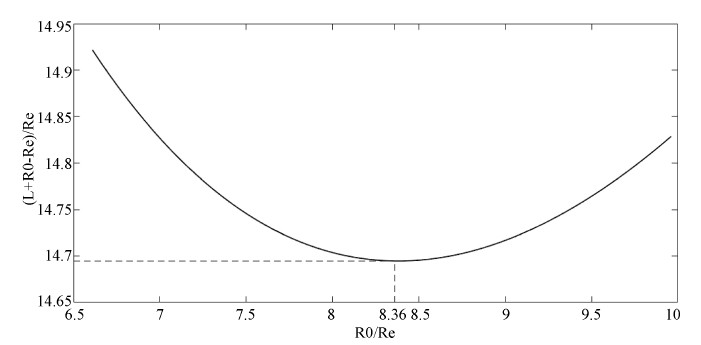Figure 3. The relationship between the length of the whole elevator and the location of the space station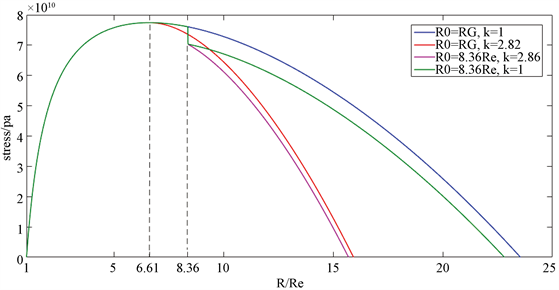Figure 4. The stress distribution of the space elevator

4. 电梯运送载荷的特性分析

4.1. 单位质量载荷运送至太空站所需能量的计算

$\Delta {\phi }_{1}+\Delta {\phi }_{2}=\frac{GM}{{R}_{e}}-\frac{GM}{{R}_{0}}-\frac{1}{2}{\omega }^{2}\left({R}_{0}^{2}-{R}_{e}^{2}\right)$ (12)

${W}_{\mathrm{min}}=\frac{GM}{{R}_{e}}-\frac{GM}{{R}_{0}}-\frac{1}{2}{\omega }^{2}\left({R}_{0}^{2}-{R}_{e}^{2}\right)$ (13)

4.2. 电梯运送载荷时太空站控制所需冲量

$I=\underset{t\left({R}_{e}\right)}{\overset{t\left({R}_{0}\right)}{\int }}\frac{r-{R}_{e}}{{R}_{0}-{R}_{e}}\cdot 2\omega v\text{d}t=2\omega \underset{{R}_{e}}{\overset{{R}_{0}}{\int }}\frac{r-{R}_{e}}{{R}_{0}-{R}_{e}}\text{d}r=\omega \left({R}_{0}-{R}_{e}\right)$ (14)

5. 结论

1) 通过增加太空电梯配重段的线密度，可以减小电梯的总长度；

2) 对于一定的电梯材料线密度比，在比地球同步轨道更高的轨道上，存在一个太空站的位置，使得电梯总长度最小；

3) 在地球同步轨道高度的位置，太空电梯所受到的拉力最大；对于碳纳米管材料，该处的应力约为77.5 GPa；

4) 载荷运送至太空站所需能量以及运送过程中太空站所需要的控制冲量，仅与太空站的位置有关，且太空站轨道高度越低，所需的控制冲量越小。

Study on Mechanical Characteristics of Space Elevator[J]. 国际航空航天科学, 2018, 06(03): 38-45. https://doi.org/10.12677/JAST.2018.63005

1. 1. Cohen, S.S. and Misra, A.K. (2007) Elastic Oscillations of the Space Elevator Ribbon. Journal of Guidance Control and Dynamics, 30, 1711-1717. https://doi.org/10.2514/1.29010

2. 2. Cohen, S.S. and Misra, A.K. (2009) The Effect of Climber Transit on the Space Elevato Dynamics. Acta Astronautica, 64, 538-553.

3. 3. Woo, P. and Misra, A.K. (2014) Energy Considerations in the Partial Space Elevator. Acta Astronautica, 99, 78-84. https://doi.org/10.1016/j.actaastro.2014.02.013

4. 4. Ishikawa, Y., Otsuka, K., Yamagiwa, Y. and Doi, H. (2018) Effects of Ascending and Descending Climbers on Space Elevator Cable Dynamics. Acta Astronautica, 145, 165-173. https://doi.org/10.1016/j.actaastro.2018.01.031

5. 5. Knapman, J.M. and Swan, P.A. (2014). Design Concepts for the First 40 km a Key Step for the Space Elevator. Acta Astronautica, 104, 526-530. https://doi.org/10.1016/j.actaastro.2014.06.004

6. 6. Quine, B.M., Seth, R.K. and Zhu, Z.H. (2009) A Free-Standing Space Elevator Structure: A Practical Alternative to the Space Tether. Acta Astronautica, 65, 365-375. https://doi.org/10.1016/j.actaastro.2009.02.018

7. 7. 李蒙, 陈伟跃, 杨胜, 等. 月球天梯力学特性研究[J]. 中国空间科学技术, 2016, 36(3): 63-69.

8. 8. 宋海蓝, 蒋婷婷, 周莉, 等. 月球天梯系统模型建立和太空舱运动的能耗计算[J]. 机械管理开发, 2018(4): 1-4.

9. 9. 汪小卫, 鲁宇, 刘丙利, 等. 天梯技术研究进展[J]. 导弹与航天运载技术, 2015(2): 41-44.

10. 10. 张普卓, 汪小卫, 刘丙利, 等. 天梯系统指标初步研究[J]. 导弹与航天运载技术, 2016(3): 5-8.

11. 11. 刘剑洪, 吴双泉, 何传新, 等. 碳纳米管和碳微米管的结构、性质及其应用[J]. 深圳大学学报理工版, 2013, 30(1): 1-11.